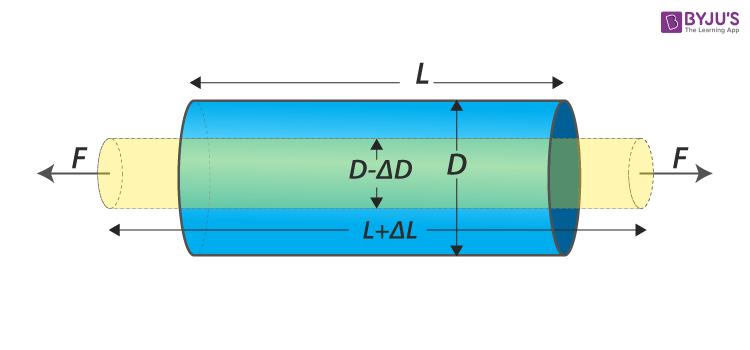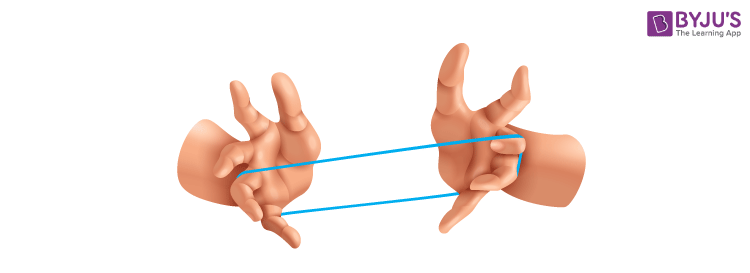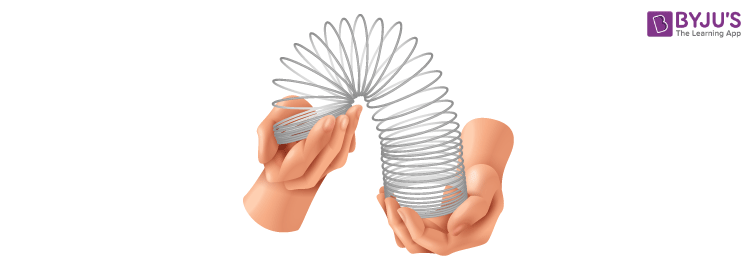# Longitudinal Strain

Have you ever wondered why objects change shape when subjected to external forces? Or how bridges and buildings can withstand the forces exerted on them? The answer lies in a fundamental concept called longitudinal strain. Longitudinal strain refers to the deformation in a material when subjected to an applied force or stress in the same direction as its original length. In this article, we will explore what longitudinal strain is, how it is calculated using a simple formula, the units used to measure it, and its significance in understanding the behaviour of materials.

## What is a Longitudinal Strain?

Strain is defined as the measure of deformation encountered by an object in the direction of the force exerted. It quantifies how much an object changes in shape or size when subjected to external forces. There are two primary types of strain: longitudinal strain and lateral strain.

Strain specifically focuses on the deformation that occurs along the length of an object when an external force is applied parallel to its original direction. It measures the change in length relative to the object’s initial length. This type of strain is denoted by the Greek letter epsilon (𝜺). In contrast, lateral strain refers to the deformation that occurs perpendicular to the applied force, causing the object to expand or contract in its transverse directions.

Longitudinal Strain Definition: Longitudinal strain is the measure of deformation experienced by an object in the direction of the applied force, relative to its original length.## Longitudinal Strain Formula

The formula for calculating longitudinal strain involves determining the change in length (ΔL) and dividing it by the original length (L) of the object. The resulting value represents the longitudinal strain experienced by the object. This information is vital in understanding how materials respond to stress and allows engineers to design structures that can withstand and accommodate such deformations.

$$\begin{array}{l}Longitudinal\,Strain=\frac{Change\;in\;the\;length}{Original\;Length}\end{array}$$

$$\begin{array}{l}\varepsilon =\frac{\Delta L}{L}\end{array}$$

L is the original length, ΔL change in length

### Longitudinal Strain Unit

Longitudinal strain is dimensionless since it is a ratio that does not have a specific unit associated with it. Instead, it is expressed as a decimal or a percentage. For example, a longitudinal strain of 0.2 indicates a 20% change in length, while a longitudinal strain of 0.5 represents a 50% change in length.

Explanantion:
The formula for longitudinal strain is ΔL / L, where ΔL represents the change in length and L represents the original length of the object. Both ΔL and L are measured in meters (m). When we divide ΔL by L in the formula, the meters (m) in the numerator and denominator cancel each other out. The result is a ratio, which does not have any units associated with it.

## Examples of Longitudinal Strain

To understand longitudinal strain better, let’s explore a few examples:

### Stretching a Rubber BandYou can observe longitudinal strain in action when you stretch a rubber band. The rubber band elongates as you apply a pulling force, demonstrating how the material responds to the applied force in the same direction as its original length.

### Compression of a SpringCompressing a spring showcases longitudinal strain as well. As you press a spring together, it shortens in length due to the applied compressive force. The material of the spring experiences longitudinal strain as it deforms under compression.

### Expansion of Metal StructuresMetal structures, such as bridges or rail tracks, expand and contract with temperature changes. This expansion and contraction result in longitudinal strain, causing the metal to elongate or contract.

These examples highlight how longitudinal strain occurs in everyday objects and relates to the deformation they experience.

## Factors Affecting Longitudinal Strain

Several factors influence longitudinal strain in materials. Understanding these factors helps explain why objects deform differently under various conditions. Here are some important factors that affect longitudinal strain:

### Material Properties

Different materials have varying properties that influence their deformation behaviour. Factors such as elasticity, stiffness, and Young’s modulus determine how much a material can stretch or compress when subjected to an applied force.

### Magnitude and Direction of Applied Forces

The magnitude and direction of applied forces impact the amount and type of deformation experienced by an object. Objects can elongate or compress depending on the nature and intensity of the applied forces.

### Temperature

Temperature can affect the behaviour of materials and their response to external forces. Thermal expansion and contraction can contribute to changes in longitudinal strain. Some materials expand or contract significantly with temperature variations, affecting their deformation characteristics.

 Related Articles:

## Applications of Longitudinal Strain

Here are a few notable applications of longitudinal strain:

### Structural Engineering

Longitudinal strain plays a vital role in designing and analysing structures like bridges, buildings, and towers. Engineers use longitudinal strain measurements to ensure that materials can withstand the forces they encounter during their lifespan. Engineers can make informed decisions to create safe and durable structures by understanding how materials deform under stress.

### Mechanical Systems

Longitudinal strain is crucial in mechanical systems. By measuring longitudinal strain, engineers can monitor the performance and integrity of mechanical parts, ensuring they function optimally and detect any potential failures or malfunctions.

### Material Testing

Longitudinal strain is extensively used in material testing. Longitudinal strain measurements help determine the mechanical properties of materials, such as their elasticity, stiffness, and strength. Scientists and researchers perform experiments to understand the behaviour of materials under different loads, forces, and environmental conditions.

## Exploring Key Concepts Related to Longitudinal Strain

These videos provide valuable insights into related concepts offering a comprehensive resource for readers to deepen their understanding of longitudinal strain and its associated principles.

### Factors Affecting Deformation### Exploring Material Behavior: Elasticity and Plasticity### Understanding the Stress-Strain Curve### Exploring Poisson’s Ratio: Understanding Material Deformation## Frequently Asked Questions – FAQs

Q1

### What is the difference between longitudinal strain and lateral strain?

Longitudinal strain refers to the deformation that occurs along the length of an object when subjected to a force parallel to its original direction. Conversely, lateral strain refers to the deformation that occurs perpendicular to the applied force, causing the object to expand or contract in its transverse directions.
Q2

### What is the unit of longitudinal strain?

Longitudinal strain is dimensionless since it is a ratio with no specific unit associated with it. Instead, it is expressed as a decimal or a percentage.
Q3

### Can longitudinal strain be negative?

Yes, longitudinal strain can be negative. A negative longitudinal strain indicates that the object has undergone compression or contraction along its length, resulting in a decrease in length relative to its original state.
Q4

### What are some common materials that exhibit significant longitudinal strain?

Materials such as rubber, elastic bands, springs, and metals can exhibit significant longitudinal strain. Rubber and elastic materials can stretch considerably under applied forces, while metals may experience longitudinal strain due to thermal expansion or contraction.
Q5

### What are the practical applications of understanding longitudinal strain?

Understanding longitudinal strain has several practical applications. It is crucial in structural engineering to design safe and durable structures, monitor mechanical systems for performance and maintenance, and conduct material testing to determine mechanical properties. Longitudinal strain also helps predict materials’ behaviour under different forces and environmental conditions, leading to advancements in various industries.

Stay tuned to BYJU’S and Fall in Love with Learning!

Test your knowledge on Longitudinal Strain